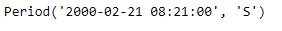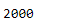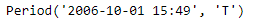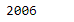Python | Pandas Period.year

• Last Updated : 06 Jan, 2019

Python is a great language for doing data analysis, primarily because of the fantastic ecosystem of data-centric python packages. Pandas is one of those packages and makes importing and analyzing data much easier.

Pandas Period.year attribute return an integer value representing the year the given period lies in.

Syntax : Period.year

Parameters : None

Return : year

Example #1: Use Period.year attribute to find the year the period lies in for the given Period object.

 # importing pandas as pdimport pandas as pd  # Create the Period objectprd = pd.Period(freq ='S', year = 2000, month = 2,                  day = 21, hour = 8, minute = 21)  # Print the Period objectprint(prd)

Output :Now we will use the Period.year attribute to find the value of year

 # return the year valueprd.year

Output :As we can see in the output, the Period.year attribute has returned 2000 indicating that the given period lies in the year of 2000.

Example #2: Use Period.year attribute to find the year the period lies in for the given Period object.

 # importing pandas as pdimport pandas as pd  # Create the Period objectprd = pd.Period(freq ='T', year = 2006, month = 10,                            hour = 15, minute = 49)  # Print the Period objectprint(prd)

Output :Now we will use the Period.year attribute to find the value of year

 # return the yearprd.year

Output :As we can see in the output, the Period.year attribute has returned 2006 indicating that the given period lies in the year of 2006.

My Personal Notes arrow_drop_up